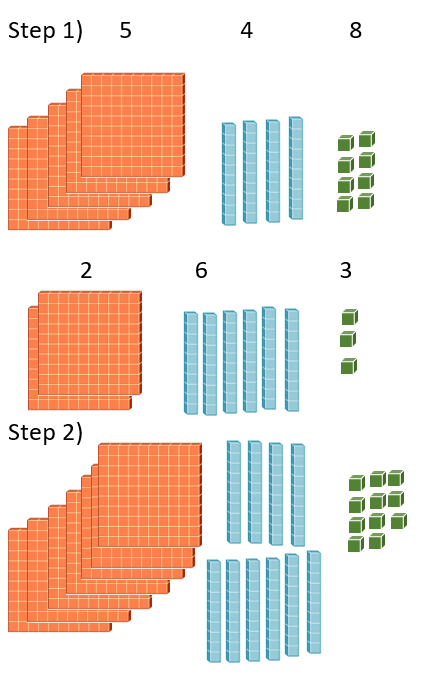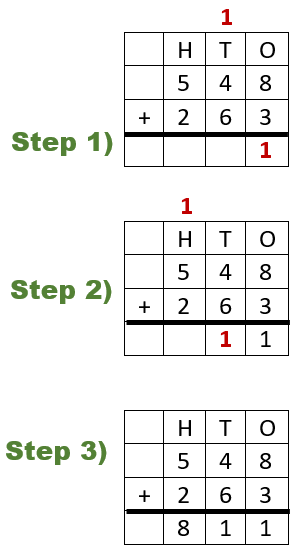# Math Worksheets Land

Math Worksheets For All Ages

# Math Worksheets Land

Math Worksheets For All Ages

Home > Math Topics > Addition >

When students progress to being able to add a pair of three-digit numbers I always recommend that you review the concept of place value. The concept of regrouping over multiple columns is often intimidating. I find that I have a much higher success rate with this part of the unit when I teach it at the beginning of the week earlier in the day. Whenever your students have the best chance attending to what you are working on, that is when you want to hit them with this. Balancing over multiple columns is one of the more difficult transitions, but I find that once you make it over this hill with students, everything is much easier as you scale up and work with much larger values. These lessons and worksheets help students learn to find the sum of triple digit numbers.

### Aligned Standard: 2.NBT.B.7

• Answer Keys - These are for all the unlocked materials above.

### Homework Sheets

These are somewhat of a single serving topic.

• Homework 1 - Well spaced and has a great deal of room to go further.
• Homework 2 - A nice and solid set of triple digits.
• Homework 3 - Equally separating these integers is key.

### Practice Worksheets

Everything follows a direct path. You will find the varying use of higher numbers to be more challenging here.

• Practice 1 - These values are tightly packed.
• Practice 2 - The comma does not appear in the final value to all them to align.
• Practice 3 - Don't get confused by what is being presented here.

### Math Skill Quizzes

These problems mimic exactly what you can expect a state assessment.

• Quiz 1 - See how well you can do these.
• Quiz 2 - What do you need to do to add these up.
• Quiz 3 - I was wishing you would look at this one last.

### Techniques to Learn Three Digit Number Addition

Now that you have come so far, playing around with the numbers and adding and subtracting them. It's time to switch things up and move to the next step. But what is the next step? Well, the next step is pretty much like the first step but with much bigger numbers. The addition is the mathematics first operation we learn about as kids. As you move up the ladder of addition, the operation becomes a little bit more complicated, and you get two and three digits to work with. If that's intimidating you, let's learn about a few things to consider when adding two-three-digit numbers.

There are 3 common strategies that are used to approach this skill. I have tried all of these techniques with my students over the years, I would encourage you to as well. While some teachers swear by one of these strategies, I have to say it completely depends on the size of your class and amount of time you have to devote to it. Here are the 3 different approaches to working this with students through solving the problem (548 + 263).Base Ten Blocks - We have used this previously when finding the sum of much smaller values. This is the same thing just on a grander scale. We would begin by converting the values into base ten blocks. We would then just put all the blocks together and count up how many there are total. When add up all the blocks in second step, we can see that there are 7 – 100s, 10 – 10s, and 11- 1s. If we have a value in any column that is 10 or greater, we carry it up to the next column, we need to do this at the lowest place value (1s) first and when it makes its way through, we on to the next place. So we can rewrite our original count as 7 – 100s, 11 – 10s, and 1- 1s. That satisfied the ones and hundreds place, but the tens place needs another go, we could rewrite this as: 8 – 100s, 1 – 10s, and 1- 1s. The final sum is therefore 811.

Breaking Apart at The Seams - This is very similar to the last technique, but it is all done with numbers. You would simply write all the values in expanded form before processing the calculation. You add the highest places and down. Going back to our original problem (548 + 263). We would rewrite it as:

500 + 40 + 8 and 200 + 60 + 3

Add hundreds: 500 + 200 = 700

Add tens: 40 + 60 = 100

Add ones: 8 + 3 = 11

Combine everything: 700 + 100 + 11 = 811

Breaking Apart at The Seams - This is very similar to the last technique, but it is all done with numbers. You would simply write all the values in expanded form before processing the calculation. You add the highest places and down. Going back to our original problem (548 + 263). We would rewrite it as:

500 + 40 + 8 and 200 + 60 + 3

Add hundreds: 500 + 200 = 700

Add tens: 40 + 60 = 100

Add ones: 8 + 3 = 11

Combine everything: 700 + 100 + 11 = 811Vertical Setup - You would place one addend directly above the other. You would then add from the lowest place value to the largest. In our problem we start at the ones place (8 + 3 = 11). We drop the 1 and carry the other 1. We add the tens place (1 + 4 + 6 = 11). Again, we drop the 1 and carry the other 1. We finish off by adding the hundreds place (1 + 5 + 2 = 8). Once again, the final sum is 811.

Unlock all the answers, worksheets, homework, tests and more!
Save Tons of Time! Make My Life Easier Now

## Thanks and Don't Forget To Tell Your Friends!

I would appreciate everyone letting me know if you find any errors. I'm getting a little older these days and my eyes are going. Please contact me, to let me know. I'll fix it ASAP.